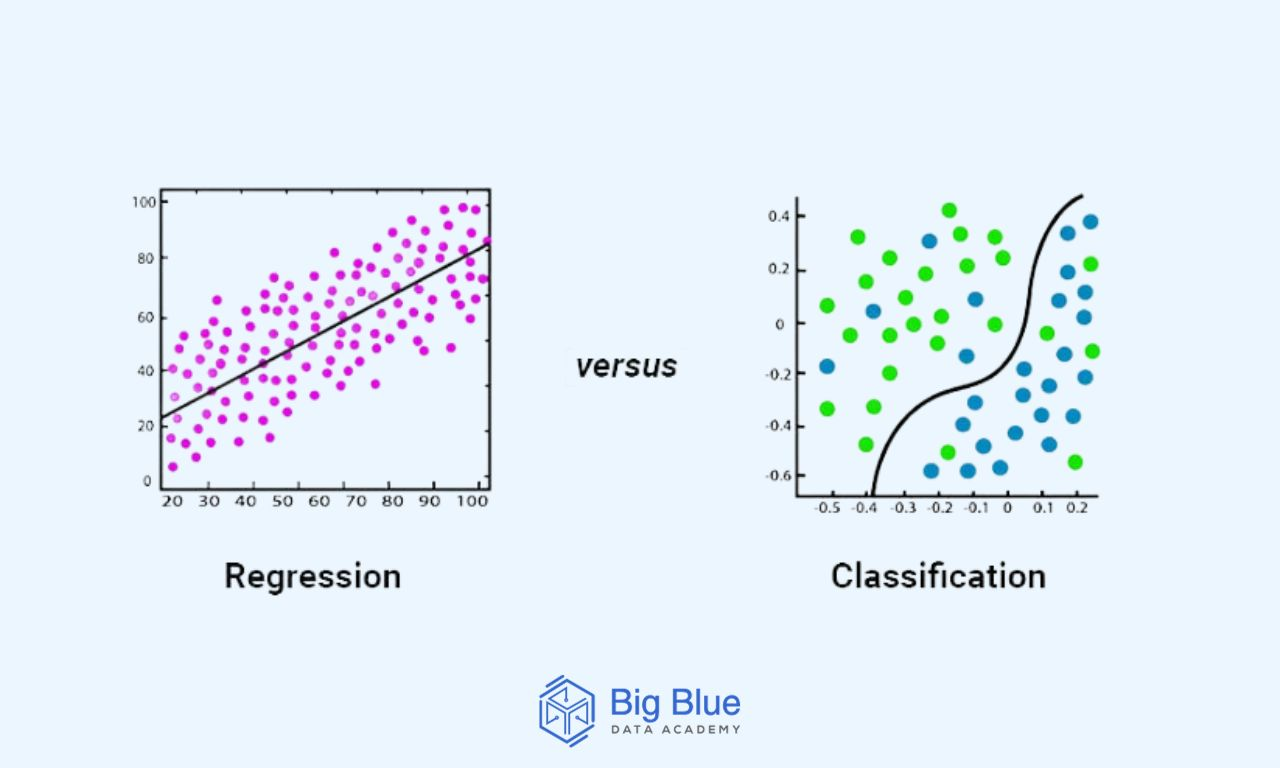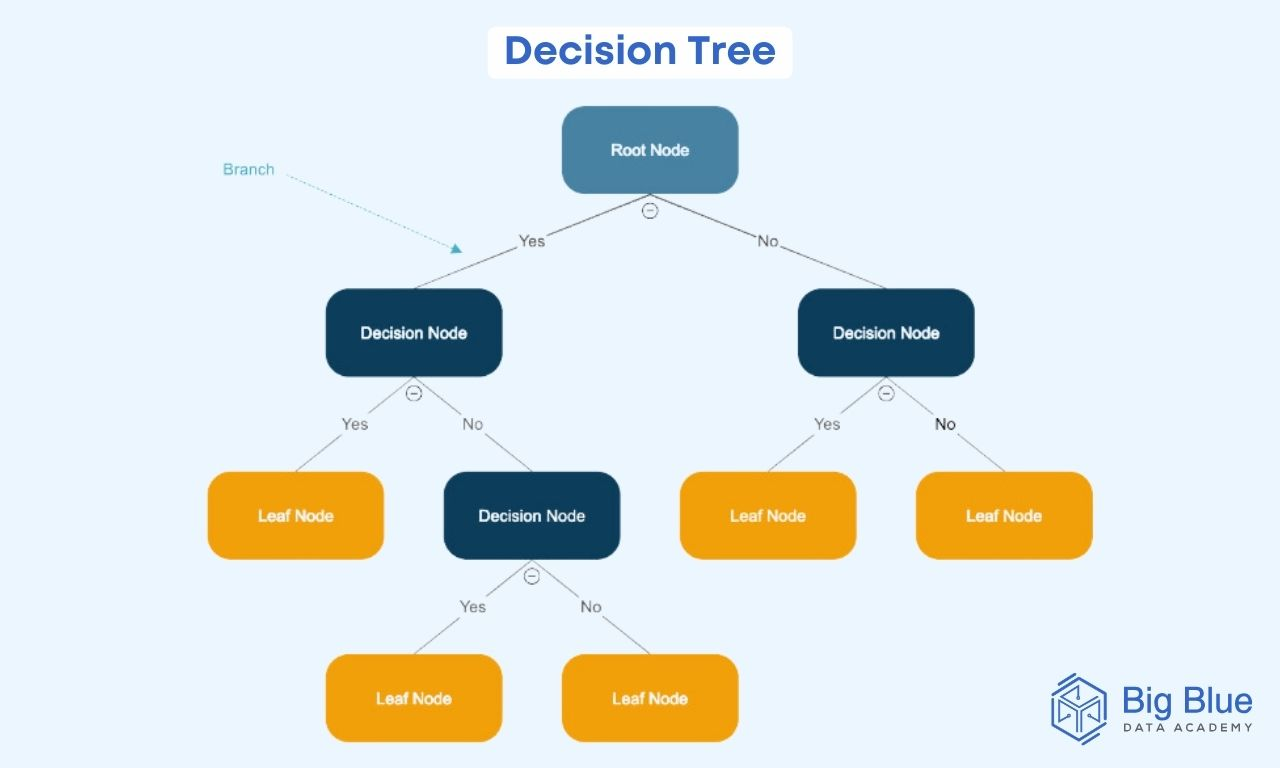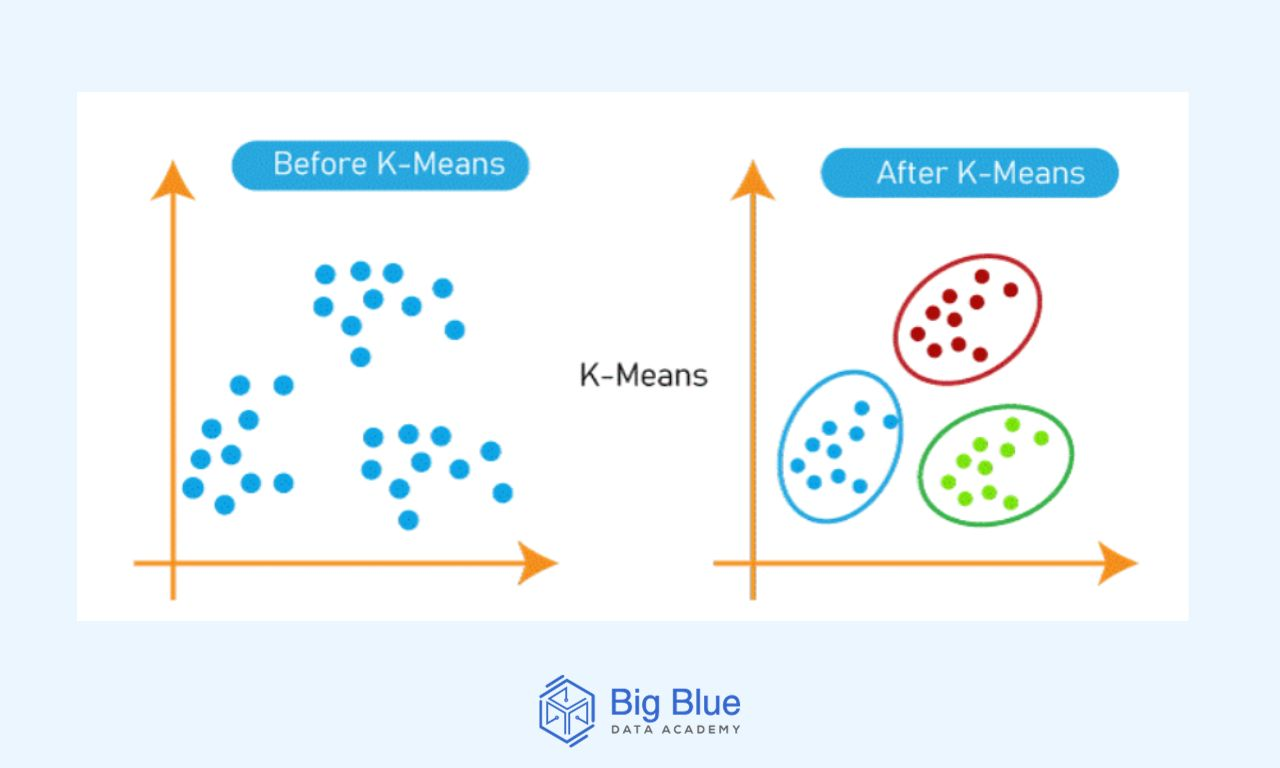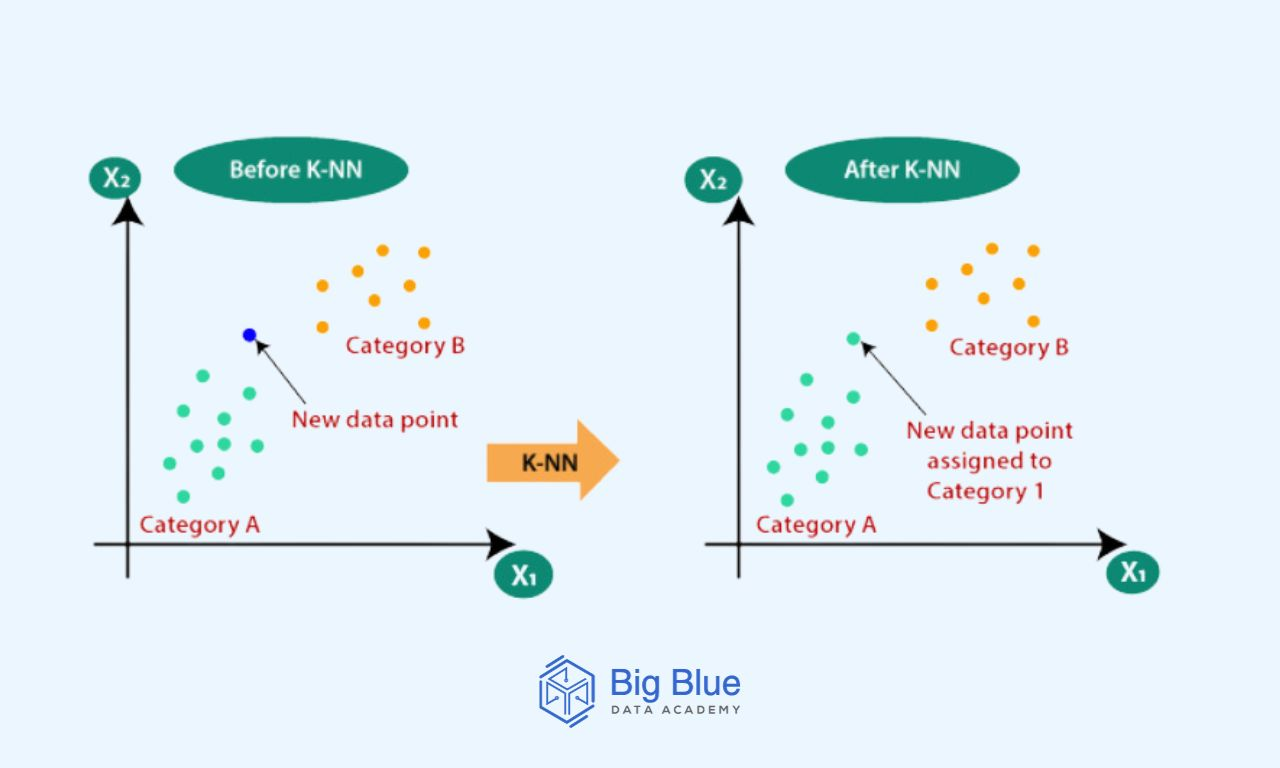# 7 Machine Learning Interview Questions (with Answers)

If you are taking your first steps in the field of machine learning and want to get informed about possible questions that may be asked in a job interview, then this guide is for you.

In today's article, we have gathered 7 basic questions that you may be asked about machine learning to help you prepare effectively and increase your chances of getting hired.

## Question #1: What Are the Differences Between Deep Learning and Machine Learning?

A fundamental question that can be asked in a machine learning interview is, of course, about the differences between deep learning and machine learning.

Let's take a look at an indicative answer:

Deep learning allows machines to make various business decisions using artificial neural networks, which is why it requires a vast amount of data for training.

In simple terms, computers learn to process data in a way inspired by the human brain.

Deep learning models can recognize complex and intricate patterns in images, text, sounds, etc., to make accurate predictions.

Machine learning, on the other hand, is a branch of artificial intelligence that enables machines to make business decisions without external assistance, using knowledge acquired from previous data.

Through machine learning, computers learn to recognize patterns in data and gradually become independent.

Also, regarding problem-solving, there is a different approach.

In machine learning, the algorithm takes the problem, breaks it into smaller pieces, and solves each one separately.

In contrast, in deep learning, the algorithm takes a problem and immediately provides the solution.

## Question #2: What Are the Differences Between Supervised and Unsupervised Machine Learning?

In supervised learning, algorithms use labeled datasets to train, where each data point is associated with a target or label.

Models receive direct feedback to confirm whether the predicted output is correct.

In supervised learning, the algorithm is trained on a labeled dataset, where each data point is associated with a target or label.

The primary goal is to train the model to predict the output when given new data.

Conversely, in unsupervised learning, algorithms use unlabeled data.

The algorithm attempts to find patterns, structures, or relationships within the data without the guidance of labeled outputs.

In unsupervised learning, the algorithm is trained on data without labels, and its goal is to discover hidden patterns or structures within the data.

Bonus point: At this point, you can also mention semi-supervised learning, which is a combination of supervised and unsupervised learning.

It is used when there is a very small labeled dataset and a large unlabeled dataset.

Essentially, the algorithm is trained on a mix of labeled and unlabeled data.

## Question #3: How Does Classification Differ from Regression in Machine Learning?

Regression and classification algorithms are both supervised learning algorithms.

Both of these algorithms are used for making predictions in machine learning and operate with labeled datasets.The main difference between regression and classification algorithms lies in the fact that regression algorithms are used for predicting continuous values, such as price and age.

On the other hand, classification algorithms are used for predicting/classifying discrete values, such as true/false, male/female, and so on.

## Question #4: What Do We Define as Decision Trees?

Decision trees are a type of supervised learning algorithm that can be used for both classification and regression problems.

As their name suggests, they are structures resembling trees, where each internal node represents a feature, branches represent decision rules, and each leaf node represents an outcome.Decision trees are known for their ease of understanding, flexibility, and the ability to solve various problems.

Additionally, decision trees can handle both categorical and numerical data.

In a decision tree, the root node (also known as the parent node) represents the entire dataset, which is divided into two or more homogeneous sets.

The leaf nodes are the final output nodes (also known as child nodes), and the tree cannot be further subdivided once a leaf node is reached.

## Question #5: What Is the Difference Between K-Means and the K-Nearest Neighbors (KNN) Algorithm?

The K-Means algorithm is an unsupervised machine learning algorithm used for clustering purposes.

K-Means helps in labeling data by forming clusters within a dataset based on the average of distances between discrete points.On the other hand, KNN (k-nearest neighbors algorithm) is a supervised, non-parametric machine learning algorithm generally used for classification and regression tasks.KNN uses proximity to classify labels or make predictions regarding the classification of an individual data point.

## Question #6: What Are Some Methods for Handling Missing Values in a Dataset?

Another question that you may be asked concerns the handling of missing values in a dataset.

Dealing with missing values is considered one of the significant challenges faced by those working in the field of data science.

Some common methods for handling missing values include:

- Deleting the specific rows.

- Replacing missing values with the mean/median/mode of the respective feature.

- Predicting the missing values using various algorithms.

- Using algorithms that support missing values.

## Question #7: What Is Overfitting in Machine Learning, and How Can It Be Avoided?

Overfitting occurs when a machine learning model provides accurate predictions for training data but not for new data.

Data scientists initially train the model on a known dataset and then use this information to predict results for new datasets.

Overfitting can happen for various reasons, such as:

- The training data size is too small and doesn't contain enough data samples to accurately represent all possible input data values

- The training data contains large amounts of irrelevant information

- The model is trained for too long on a single dataset

To avoid overfitting, a large volume of data should be used.

However, if you have a small dataset and need to create a model based on it, you can use a technique called cross-validation.

In this method, a model is typically given a set of known data for training and a set of unknown data for testing during the training phase.

The primary goal of this method is to define a dataset for "testing" the model during its training phase.

## In a Nutshell

We've seen 7 basic interview questions for machine learning, along with the corresponding answers.

This way, a data scientist can have a better understanding of the questions they may be asked and prepare more effectively for a potential interview.

If you are intrigued and want to learn more regarding machine learning, follow us and will keep you updated with more educational articles!